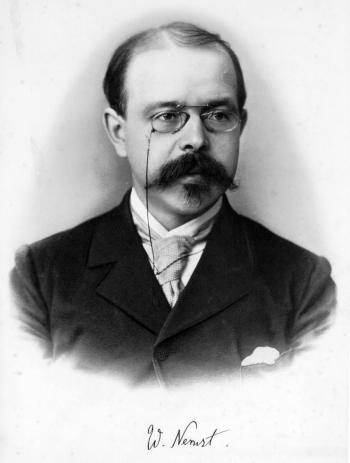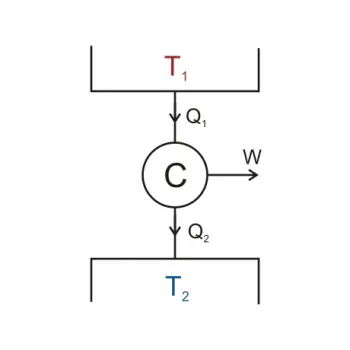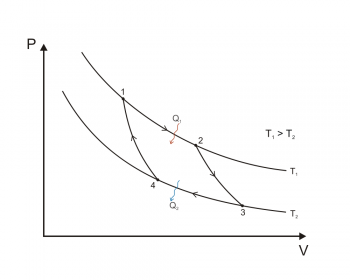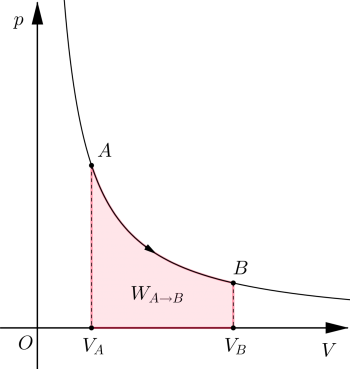# Solar EnergyPanels photovoltaic solar energyInstallation of thermal solar energySolar power plant Thermoelectric

# Entropy

## Third Law Of ThermodynamicsThe third law of thermodynamics, sometimes called Nernst's Theorem or Nernst Postulate , relates the entropy and temperature of a physical system.

The third law of thermodynamics states that absolute zero can not be achieved in a finite number of stages. The third law of thermodynamics can also be defined as that when reaching absolute zero, 0 degrees Kelvin, any process of a physical system stops and when reaching absolute zero the entropy reaches a minimum and constant value.

This principle states that the entropy of a system at the absolute zero temperature is…

+ info

## EntropyWhat is entropy? Entropy (S) is a thermodynamic quantity originally defined as a criterion for predicting the evolution of thermodynamic systems.

Entropy is a function of extensive character state. The value of entropy, in an isolated system, grows in the course of a process that occurs naturally. Entropy describes how a thermodynamic system is irreversible.

The meaning of entropy is evolution or transformation. The word entropy comes from the Greek.

Entropy in the world of physics

In physics, entropy is the thermodynamic magnitude that allows us to calculate the…

+ info

## Second Law Of ThermodynamicsAccording to the first law of thermodynamics, every process that occurs in a given system must satisfy the principle of conservation of energy, including the flow of heat.

Equation:

states, in other words, that any process whose sole purpose is to create or destroy energy, is impossible, that is, it denies the existence of a first-class perpetual motion machine.

However, the first law does not tell us anything about the…

+ info

## Thermodynamic ProcessesA thermodynamic process is the evolution of certain properties, which are called thermodynamic properties, in relation to a particular thermodynamic system. In order to study a thermodynamic process, it is required that the system be in thermodynamic equilibrium at the initial and final point of the process; that is, that the magnitudes that undergo a variation when passing from one state to another must be completely defined in their initial and final states.

In this way thermodynamic processes can be interpreted as the result of the interaction of one system with another…

+ info

## Laws Of ThermodynamicsThermodynamics is mainly based on a set of four laws that are universally valid when applied to systems that fall within the constraints implicit in each.

The first principle that was established was the second law of thermodynamics, as formulated by Sadi Carnot in 1824. The 1860 already established two "principles" of thermodynamics with the works of Rudolf Clausius and William Thomson, Lord Kelvin. Over time, these principles have become "laws." In 1873, for example, Willard Gibbs claimed that there were two absolute laws of thermodynamics in his graphical methods in fluid thermodynamics.…

+ info

## ThermodynamicsThermodynamics is the branch of classical physics that studies and describes the thermodynamic transformations induced by heat and work in a thermodynamic system, as a result of processes that involve changes in the temperature and energy state variables.

Classical thermodynamics is based on the concept of macroscopic system, that is, a portion of physical mass or conceptually separated from the external environment, which is often assumed for convenience that is not disturbed by the exchange of energy with the system. The state of a macroscopic system that is in equilibrium conditions…

+ info

## TemperatureDefinition of temperature: The temperature is the thermodynamic magnitude that shows the thermal energy of one body in relation to another.

Although the definition of temperature is simple and concise, you can explain what the temperature is in a more extensive way:

What is the temperature?

Temperature is a physical quantity of matter that quantifies the common notions of heat and cold. The objects of low temperature perceive them cold, while objects of higher temperatures we consider them warm or hot. This physiological sensation of cold and heat is generated…

+ info

## Isothermal ProcessIn thermodynamics, an isothermal process is a thermodynamic transformation at constant temperature, that is, a variation of the state of a physical system during which the temperature of the system does not change with time. Devices called thermostats can maintain a constant temperature value.

The isothermal transformation of a perfect gas is described by Boyle's law which, in a pressure-volume diagram (or Clapeyron's plane), is represented by a branch of the equilateral hyperbola.

Isotherm of a perfect gas Calculation of heat and work exchanged

For isothermal gas…

+ info

## ThermodynamicsThermodynamics is the branch of physics that studies the effects of changes in temperature, pressure and volume of a physical system (a material, a liquid, a set of bodies, etc.), at a macroscopic level. The term "thermo" means heat and dynamics refers to motion, so thermodynamics studies the movement of heat in a body. Matter is composed of different particles that move disorderly. Thermodynamics studies this disorderly movement.

The practical importance of thermodynamics lies primarily in the diversity of physical phenomena it describes. Knowledge of this diversity has resulted in…

+ info